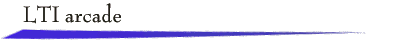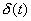Introduction

Continuous-time, dynamical systems that are linear and time-invariant, usually called LTI systems, comprise an important subclass of all systems. Several different mathematical representations are used to describe the input-output behavior of a causal LTI system, including

·        unit impulse response and convolution integral,

·        linear differential equation

·        Fourier frequency response function (stable system)

·        Laplace transfer function

LTI systems exhibit a rich variety of input-output behaviors. Characteristics of input-output behavior become important in a control systems setting, where the objective is to achieve desired output signal behaviors by applying an appropriate input signal.

The Applet

The applet permits exploration of the input-output behavior of several basic LTI systems. By selecting targets for the output signal, the applet becomes a video game. To make the game easier, the autonomous behavior of time is suspended, and you define the time scale as you sketch the input signal!

All the systems are causal, and the mathematical descriptions listed above are displayed using the notationfor the unit impulse and u(t) for the unit step function. Included are plots of the unit impulse response, unit step response, and a Bode magnitude diagram for the transfer function.

All signals are assumed to be zero for t < 0, a condition that often is made explicit by appending u(t).

Select a system and a number of targets. Then sketch an input signal x(t) so that the response y(t)  minimizes the sum-squared miss distance at the targets. To make the task more challenging, impose on youself an upperbound on the elapsed time. An equally important objective is to correlate the mathematical description of the system with the characteristics of input-output behavior.